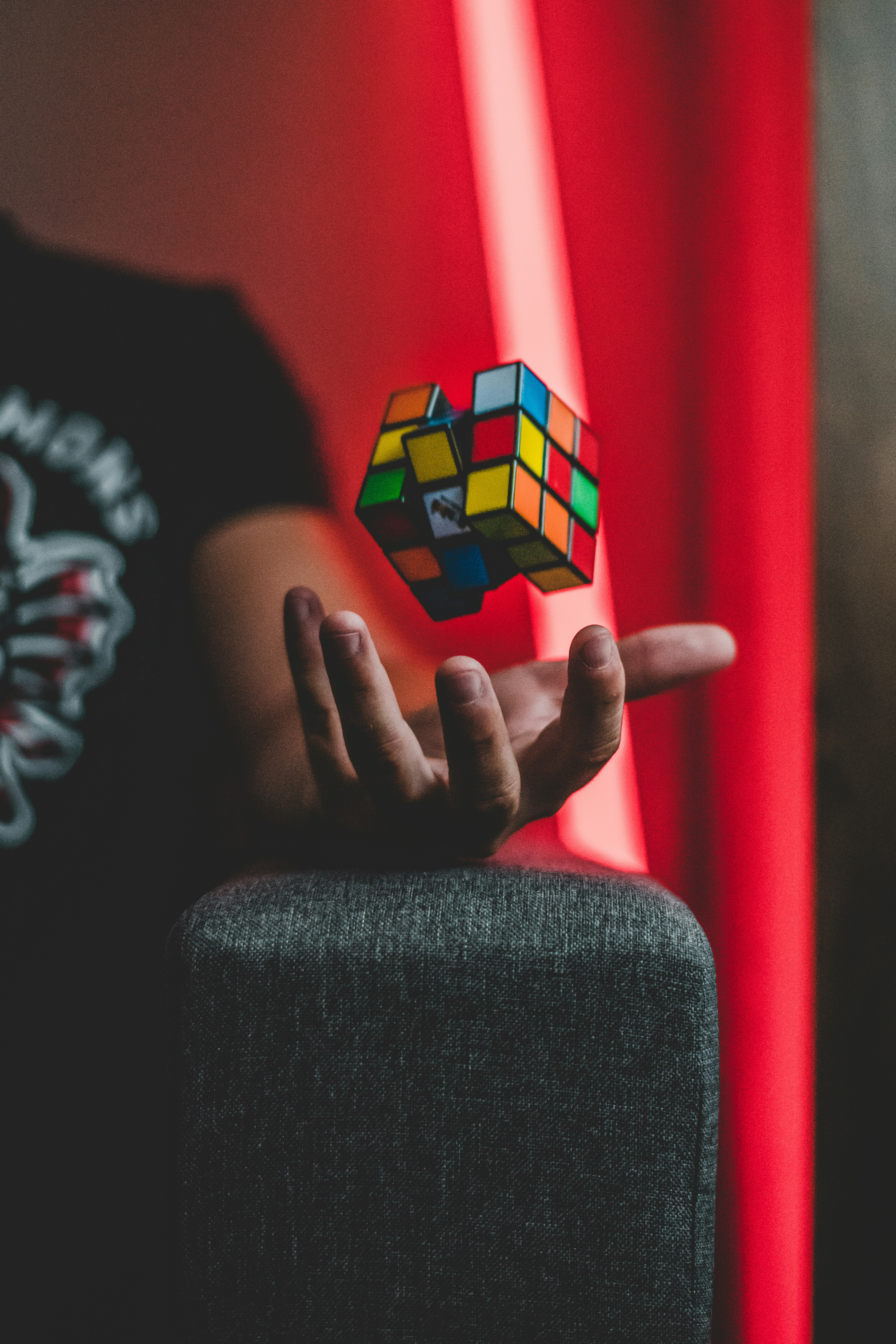Image from Marko Blažević on Unsplash

# Forces

Created by: @varun

Imported from Forces by suemercer on Blooket.

👁 Public

Tagged as: Science, Physics

History:

• Last updated last year
• Created last year

Played 0 times

Practiced 6 times

What is the name of the force that pulls all objects towards each other?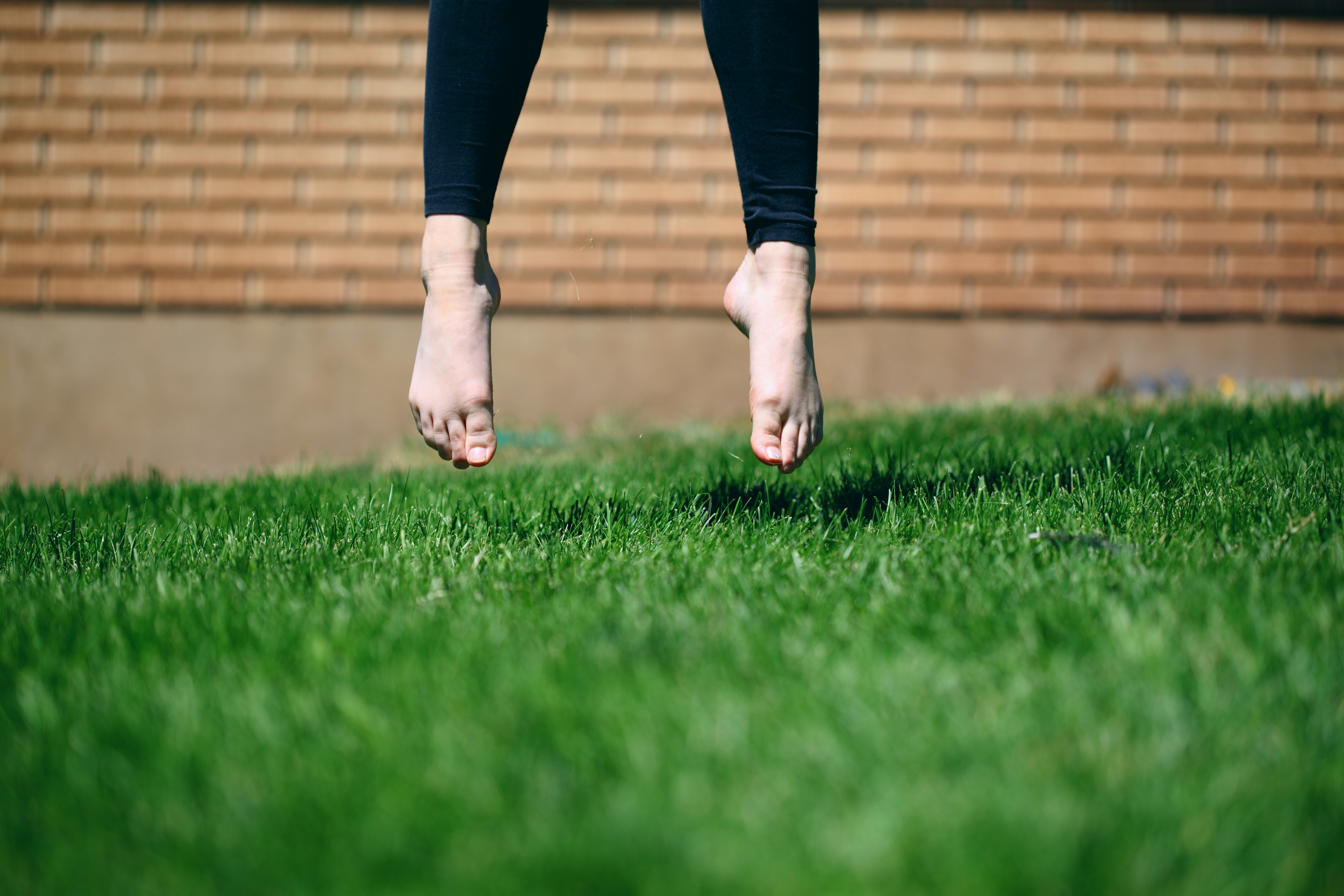Gravity
Friction
Balanced forces
Magnetic forces
The force of gravity on an object is called?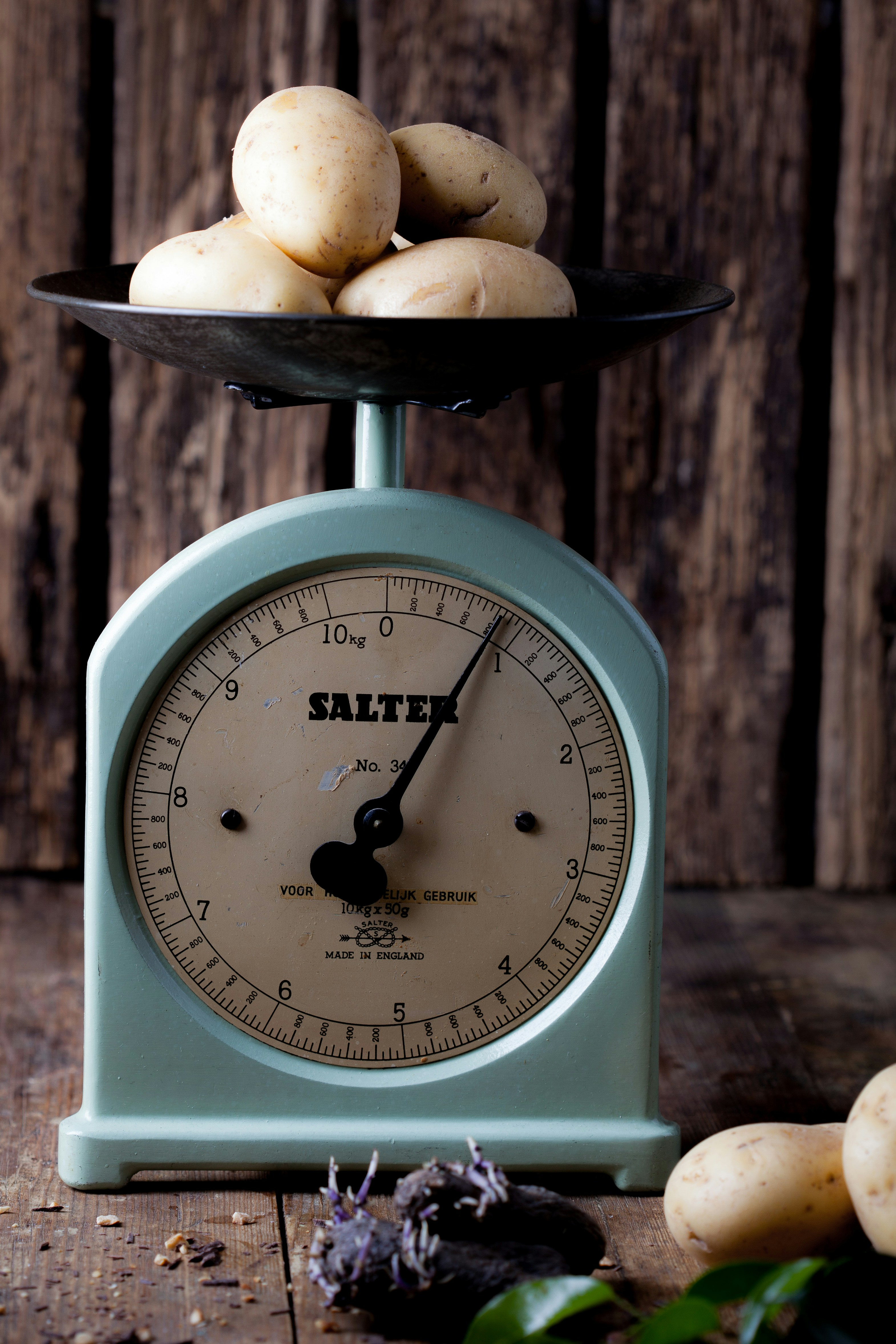Friction
Weight
Mass
Terminal Velocity
The amount of matter in an object is called?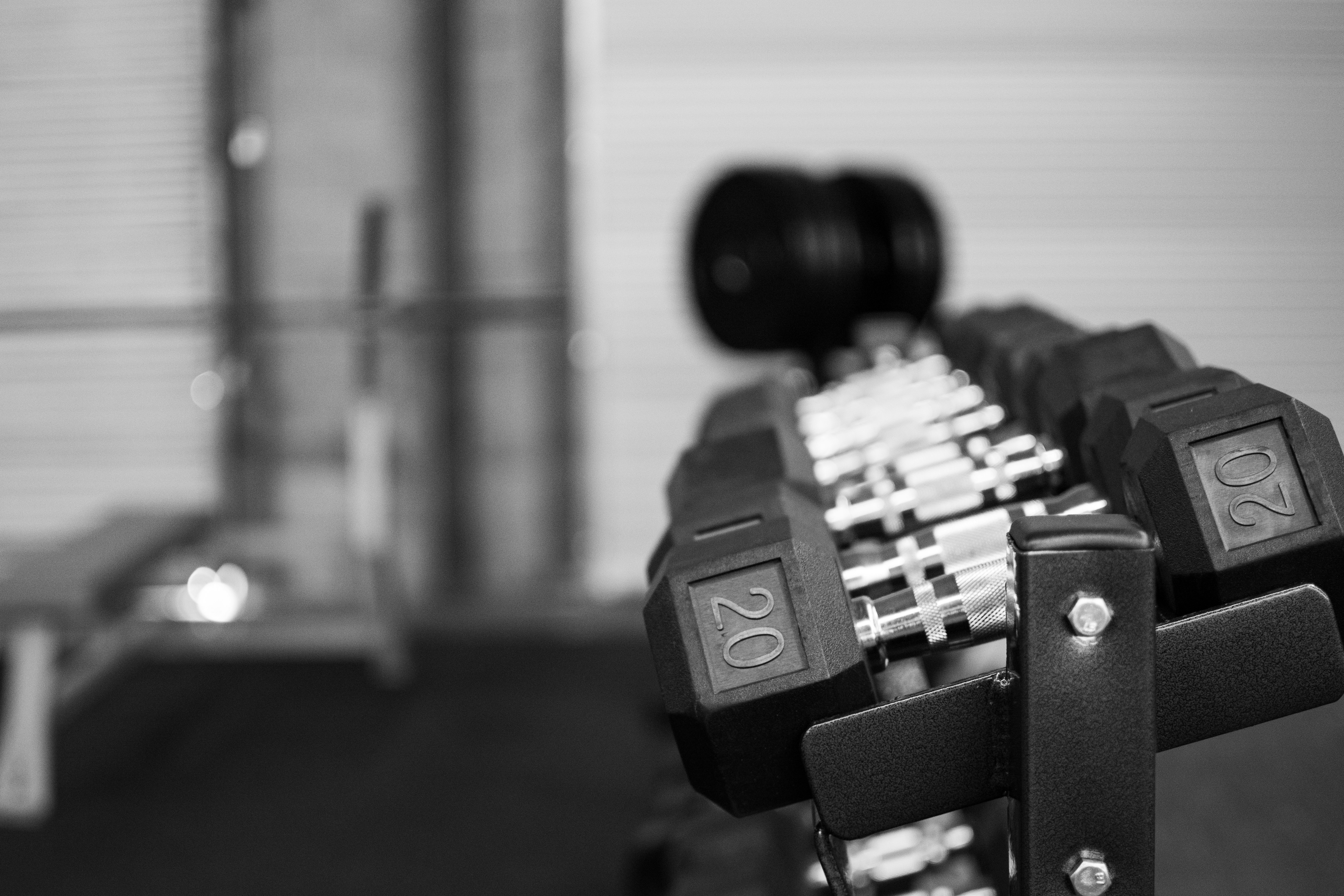gravity
mass
weight
velocity
Which type of friction is shown?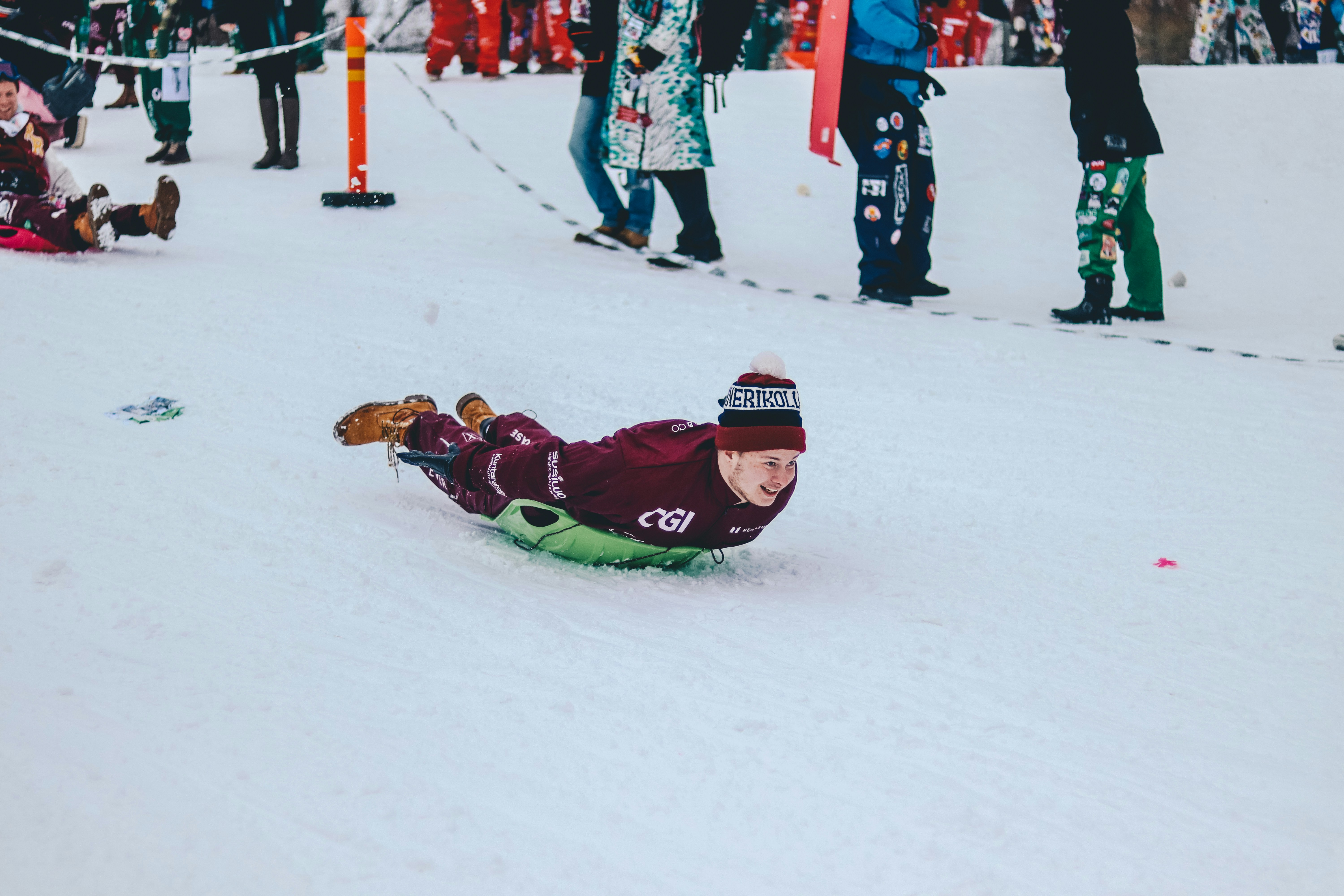fluid
rolling
gravitational
sliding
Near the surface of the earth, acceleration due to gravity is: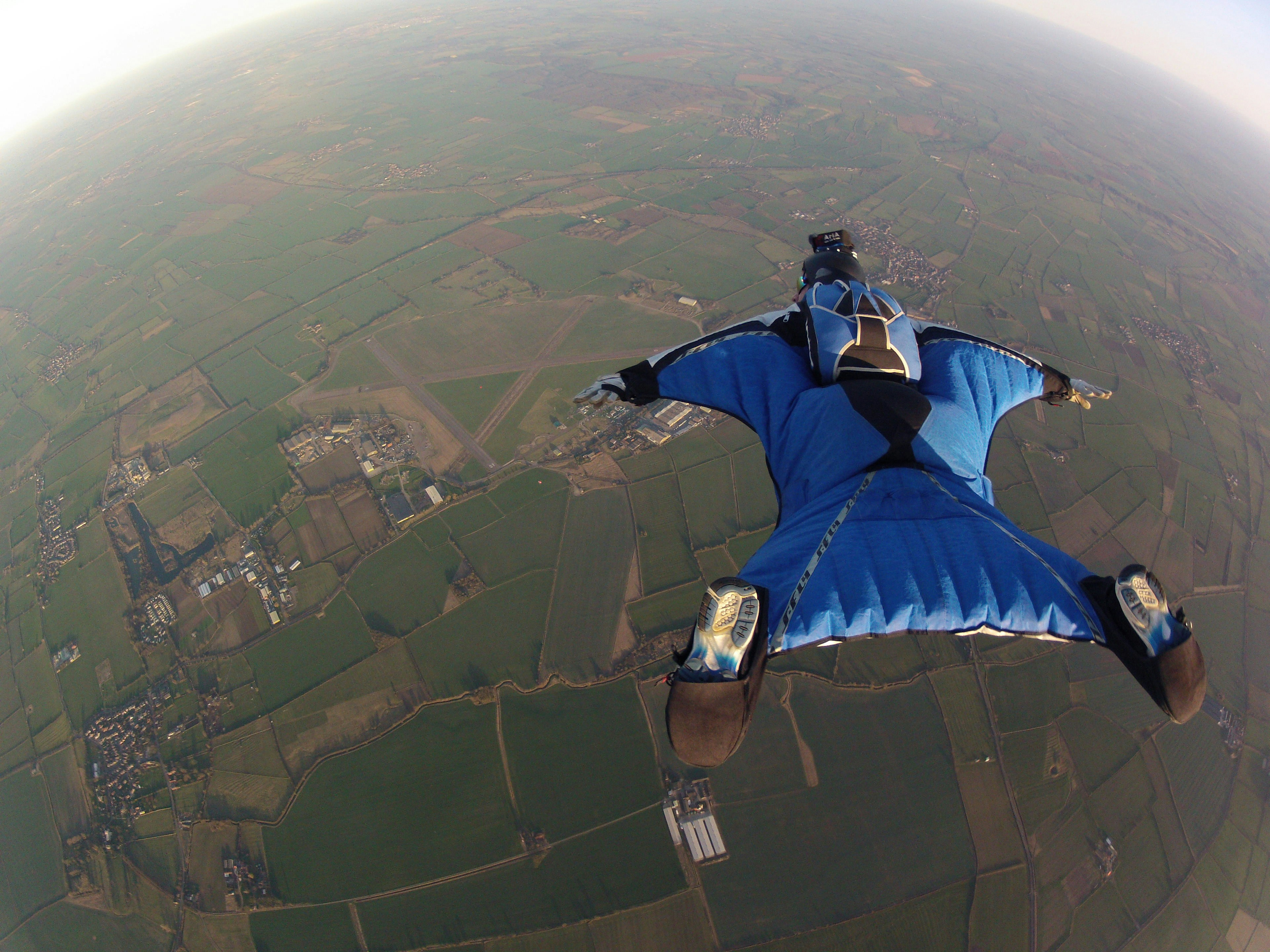98 m/sec/sec
19.8 m/sec/sec
terminal velocity
9.8 m/sec/sec
A push or a pull exerted on an object is a(n):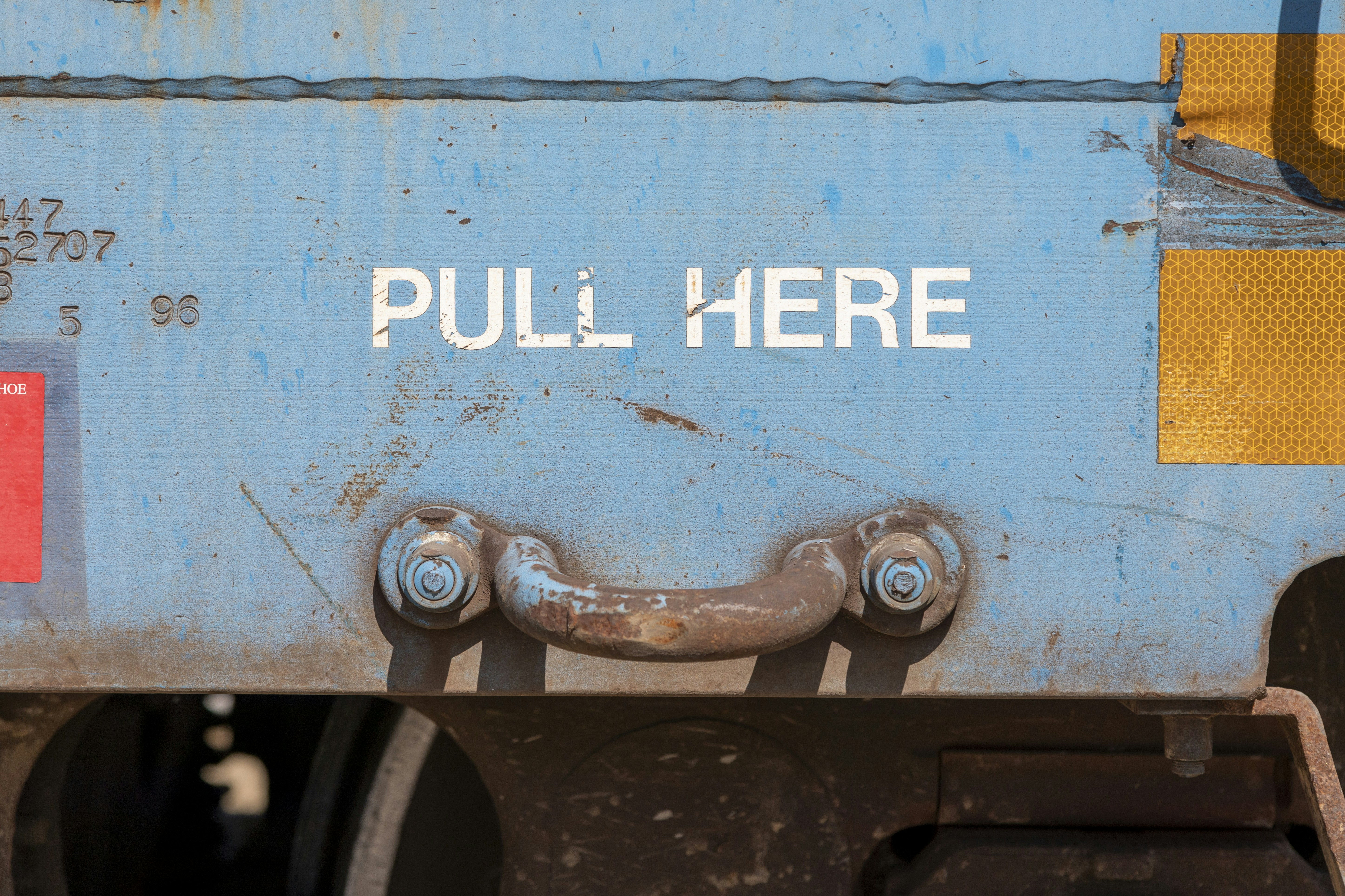force
potential energy
energy
momentum
Which of the following is the best definition for Net Force?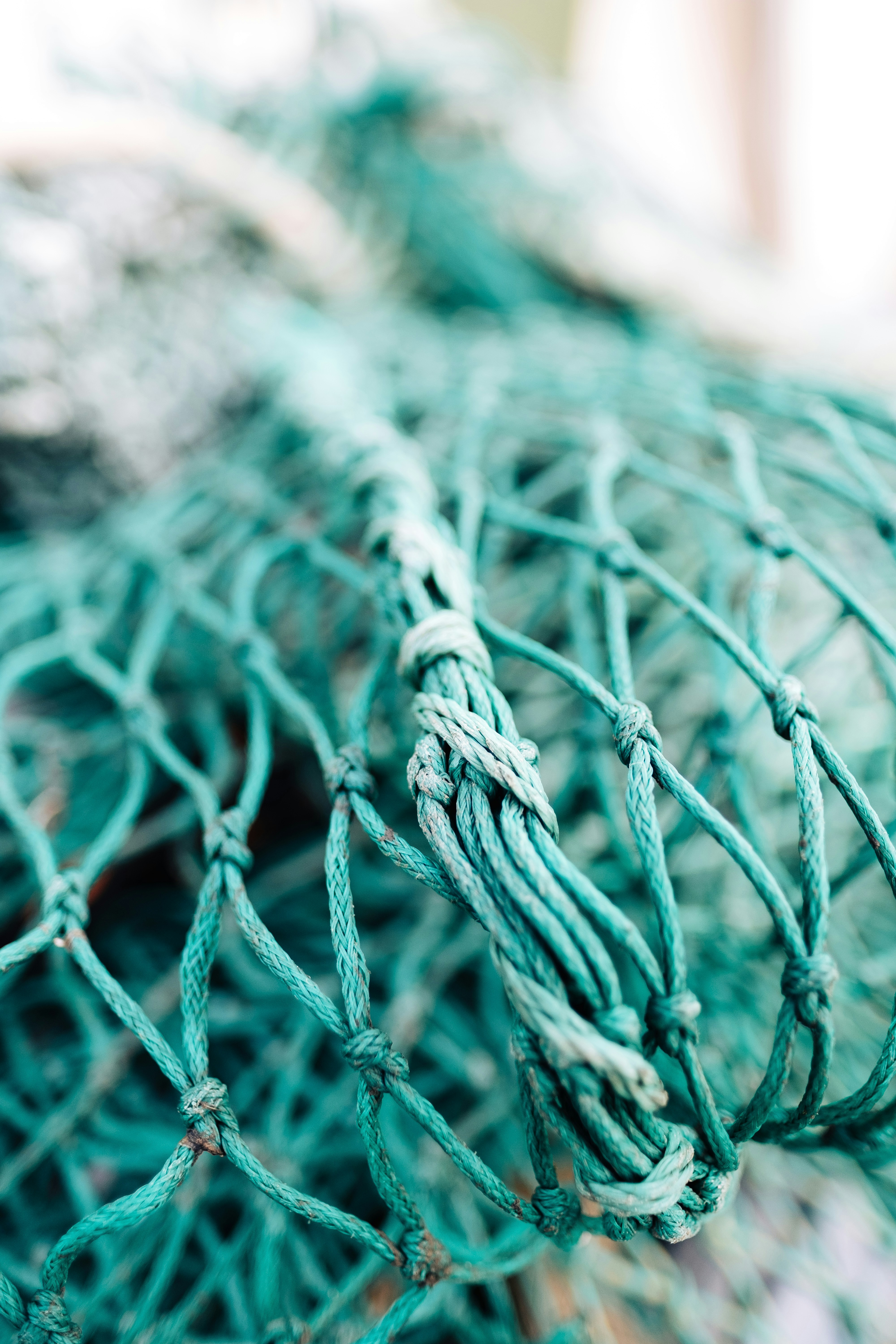The slowest speed an object can move.
The combination of all the forces acting on an object.
The combination of all the distances an object can move.
The maximum speed an object can move.
The force that two surfaces exert on each other when they rub against each other is called: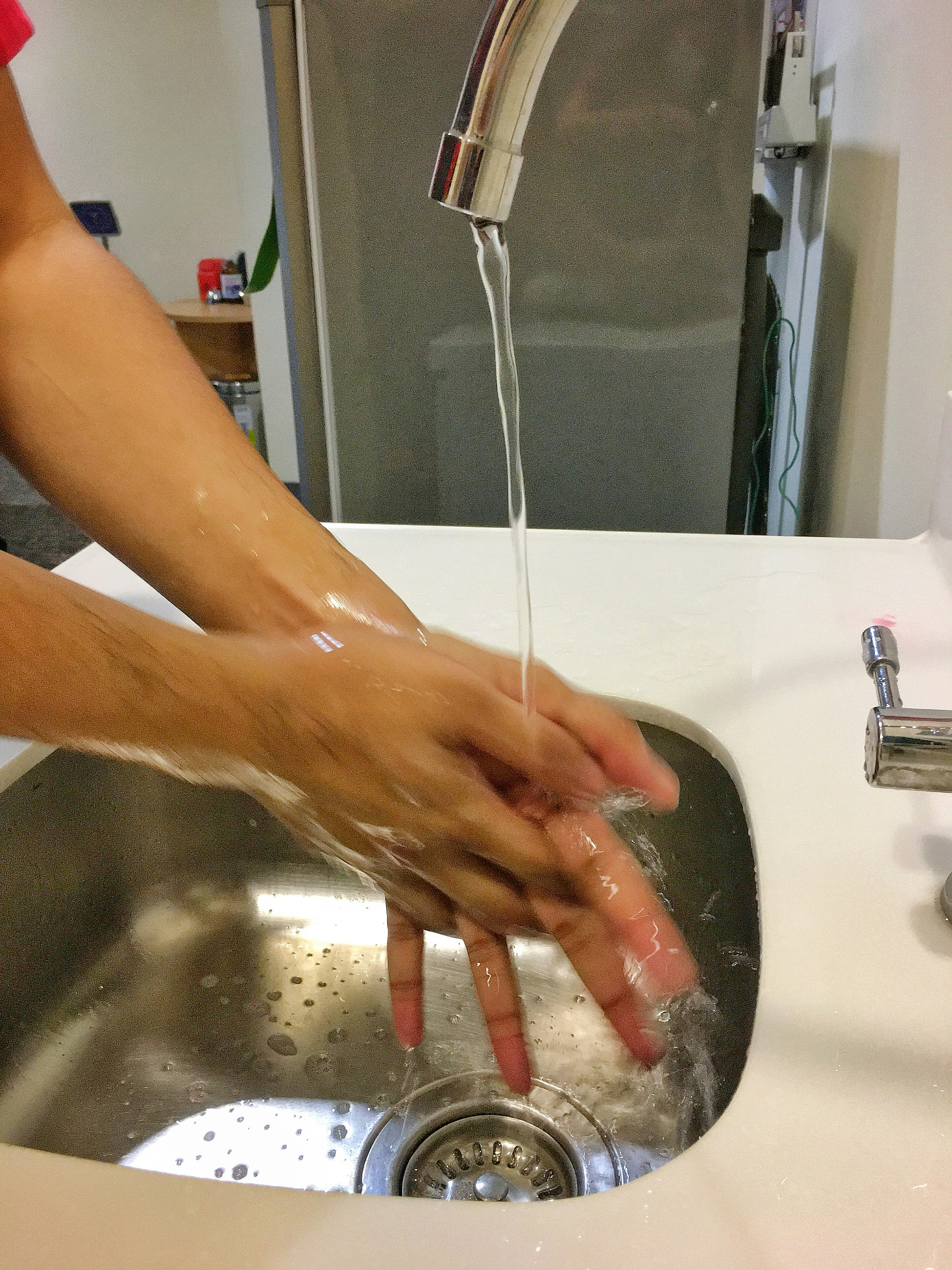Magnetism
Gravity
Friction
Net force
The strength of a force is measured in the SI unit called the: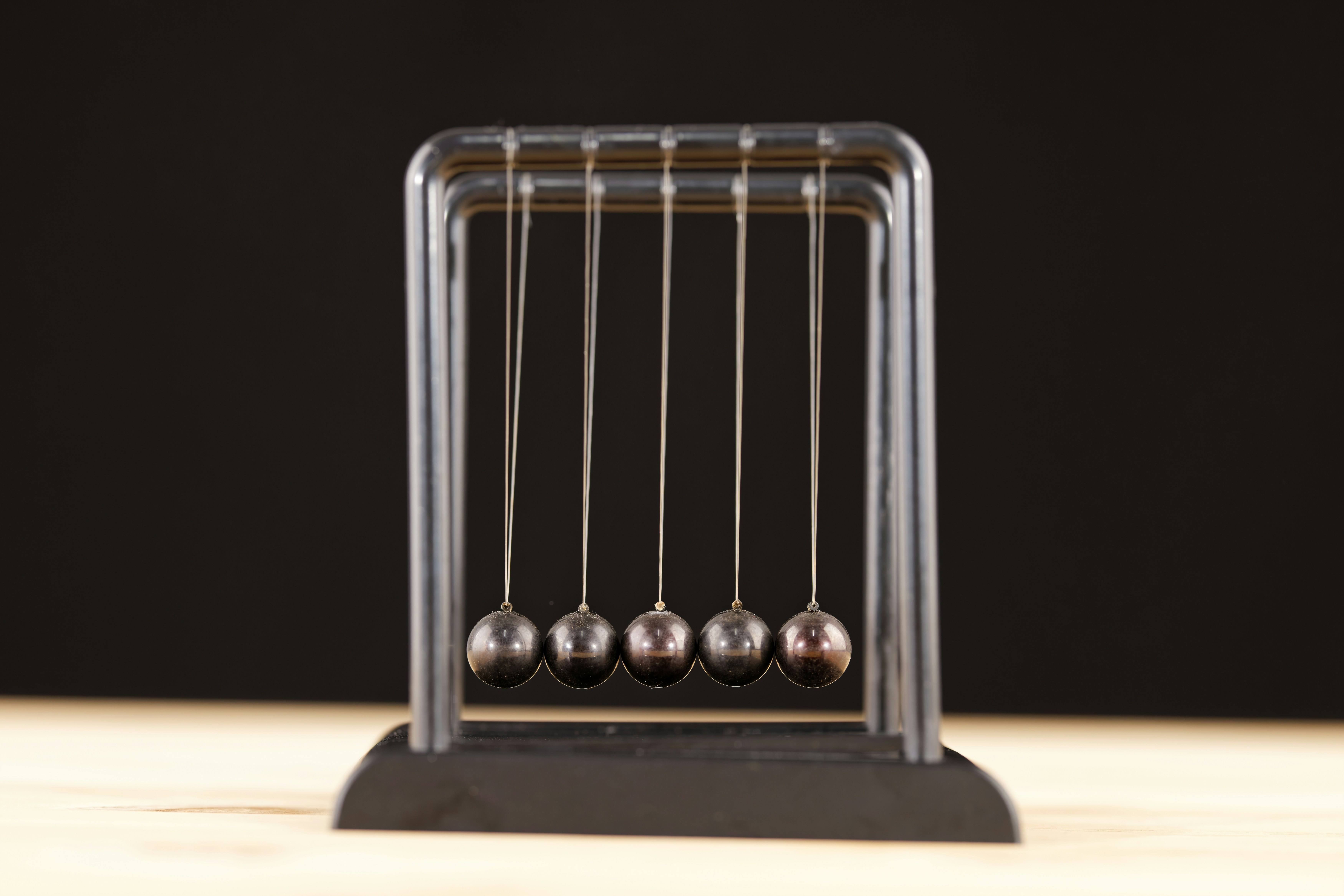Newton
Nucleus
Neutron
Net force
How do you find the net force of unbalanced forces that are in the same direction?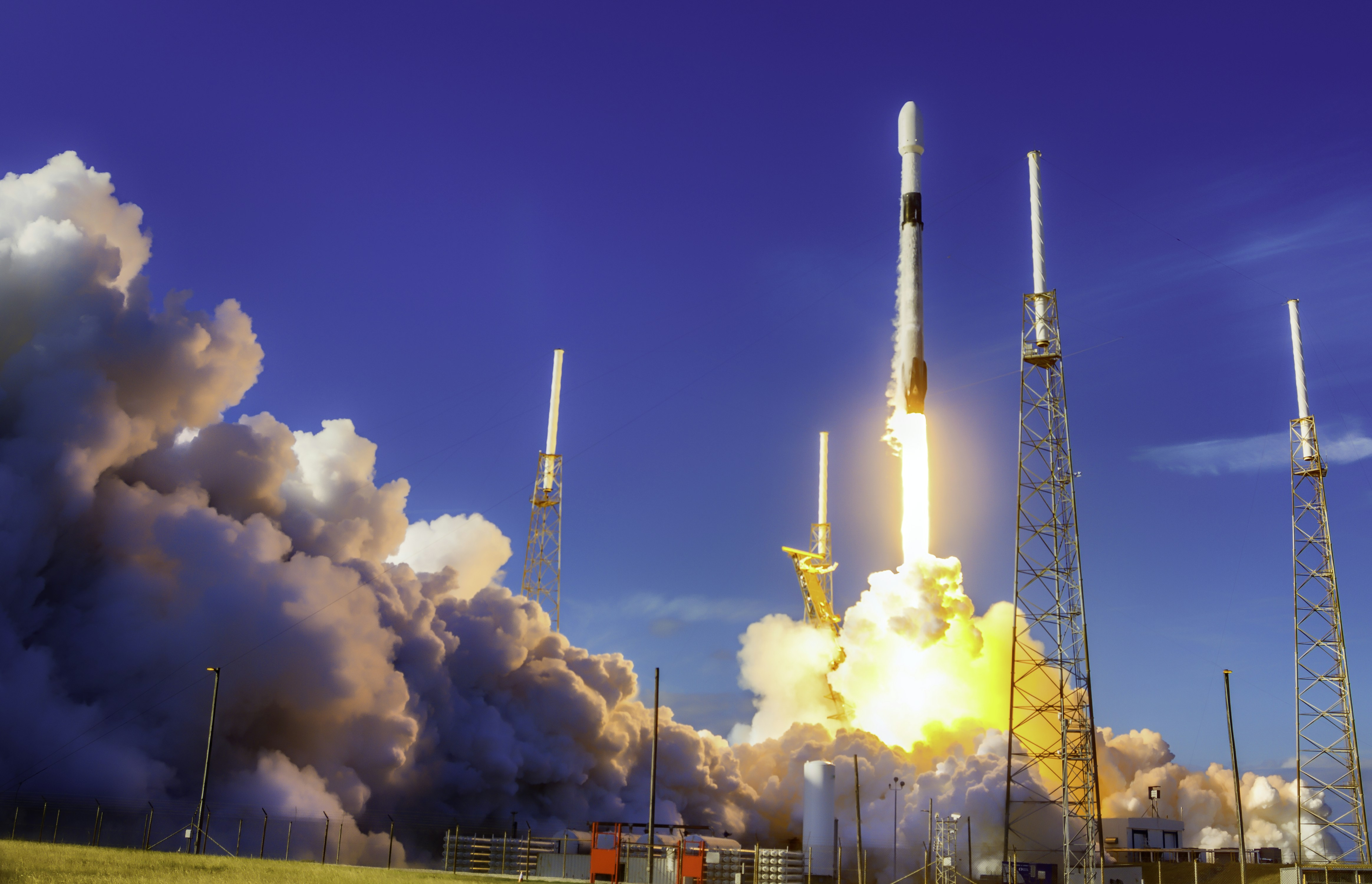Multiply them
Subtract them
Divide them
In order to make the rocket lift - off the forces must be: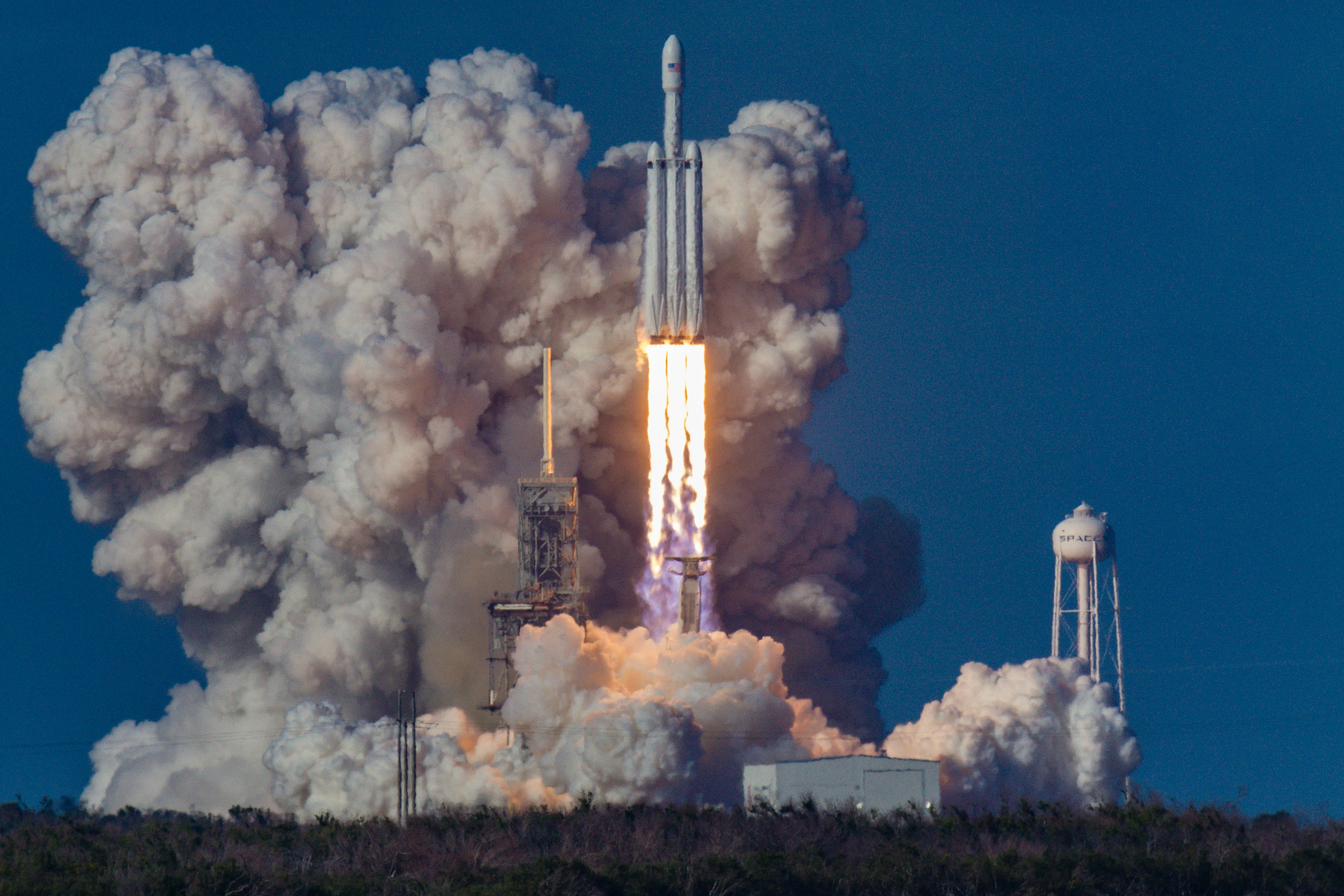balanced
equal
unbalanced
small
When balanced forces are acting on the object...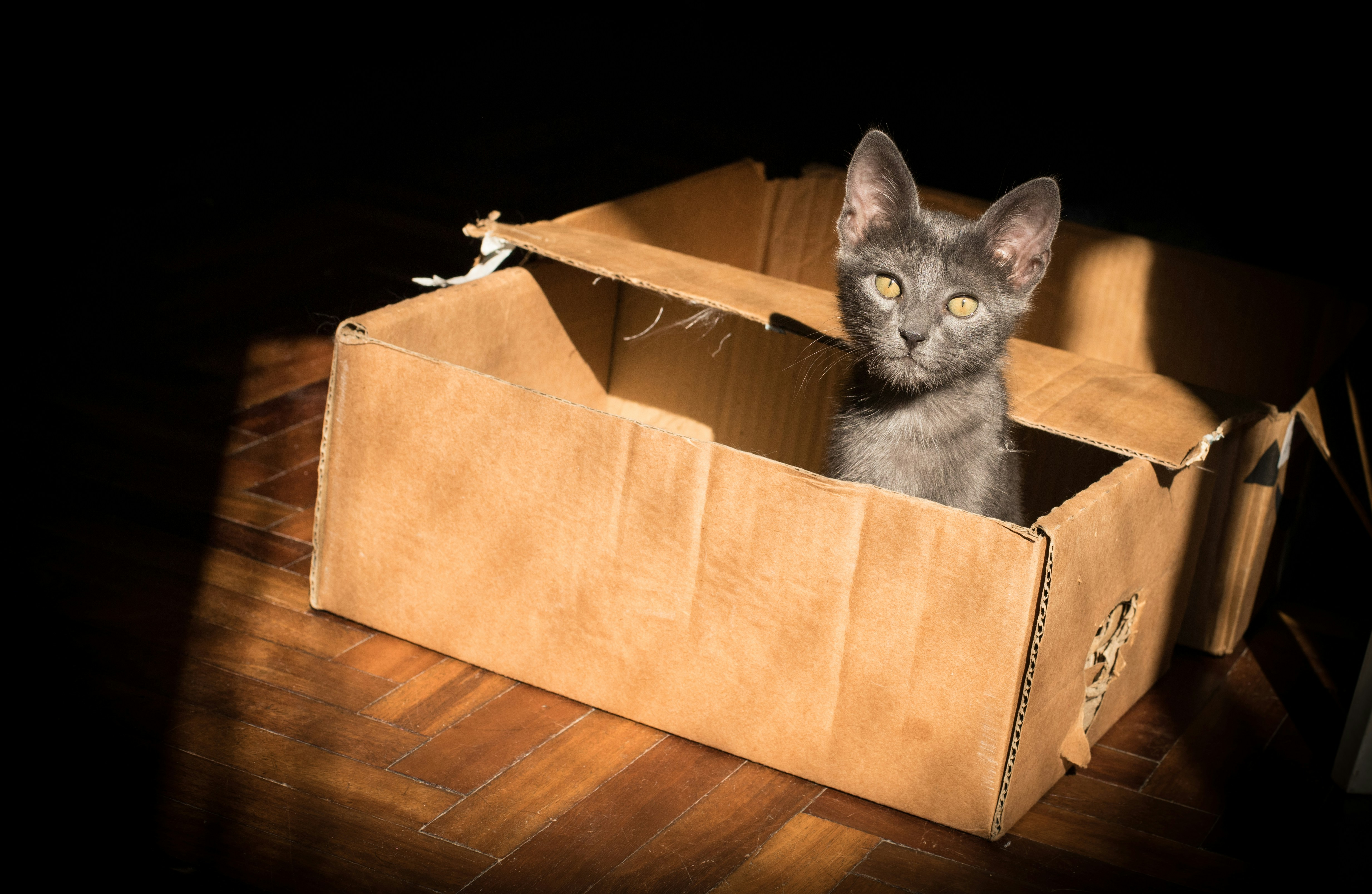The object will move.
There is not a change in motion.
The object will move very fast.
There is a change in motion.
Which of the following statements are true about weight?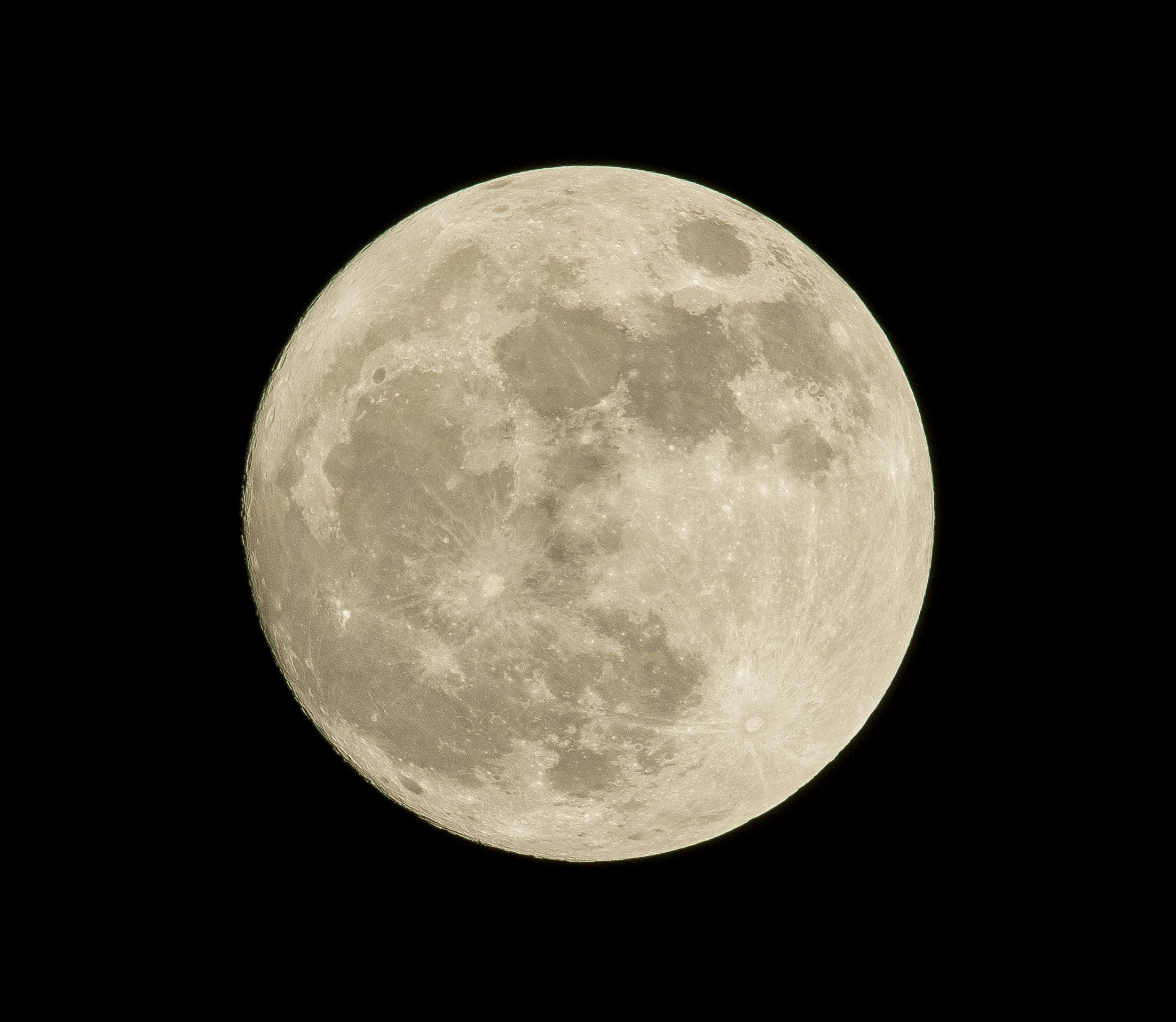your weight can change depending on the amount of gravity
weight is labeled in kilograms
weight is the amount of matter in an object
weight is the same as mass
Which type of force is shown in the picture?Frictional
Gravitational
Applied
Magnetic
Which of the following is the best description of what is happening in the picture shown?There will be movement to the right.
The forces are balanced.
There will be movement to the left.
The forces are not balanced.
Which direction will the cart move?in the directions of the blue arrows
there will not be any movement
in the direction of the red arrows
The apple falling towards the ground is an example of which type of force?Gravity
Applied
Friction
Magnetic
What is the net force?100 Newtons towards the blue
zero newtons
100 Newtons towards the red
200 Newtons
Which type of force has north and south poles which can either attract or repel each other?Applied
Frictional
Gravitational
Magnetic
Which type of force is shown in the image?balanced
terminal velocity
unbalanced
static friction
Which direction will the balloon go? There is a 5,000N force going up and 4,000N of force going down.The balloon will not move
1,000 N up
9,000 N
1,000 N down
Which type of friction is shown in the image?Fluid
Sliding
Static
Rolling
In order for the rock to move, which of the following must happen?Apply an unbalanced force.
Apply a balanced force.
Increase the air resistance.
Which type of friction does a moving sled represent?Fluid
Sliding
Static
Rolling
Friction causes an object to slow down.yes
no
Magnetic forces can attract or repel objects.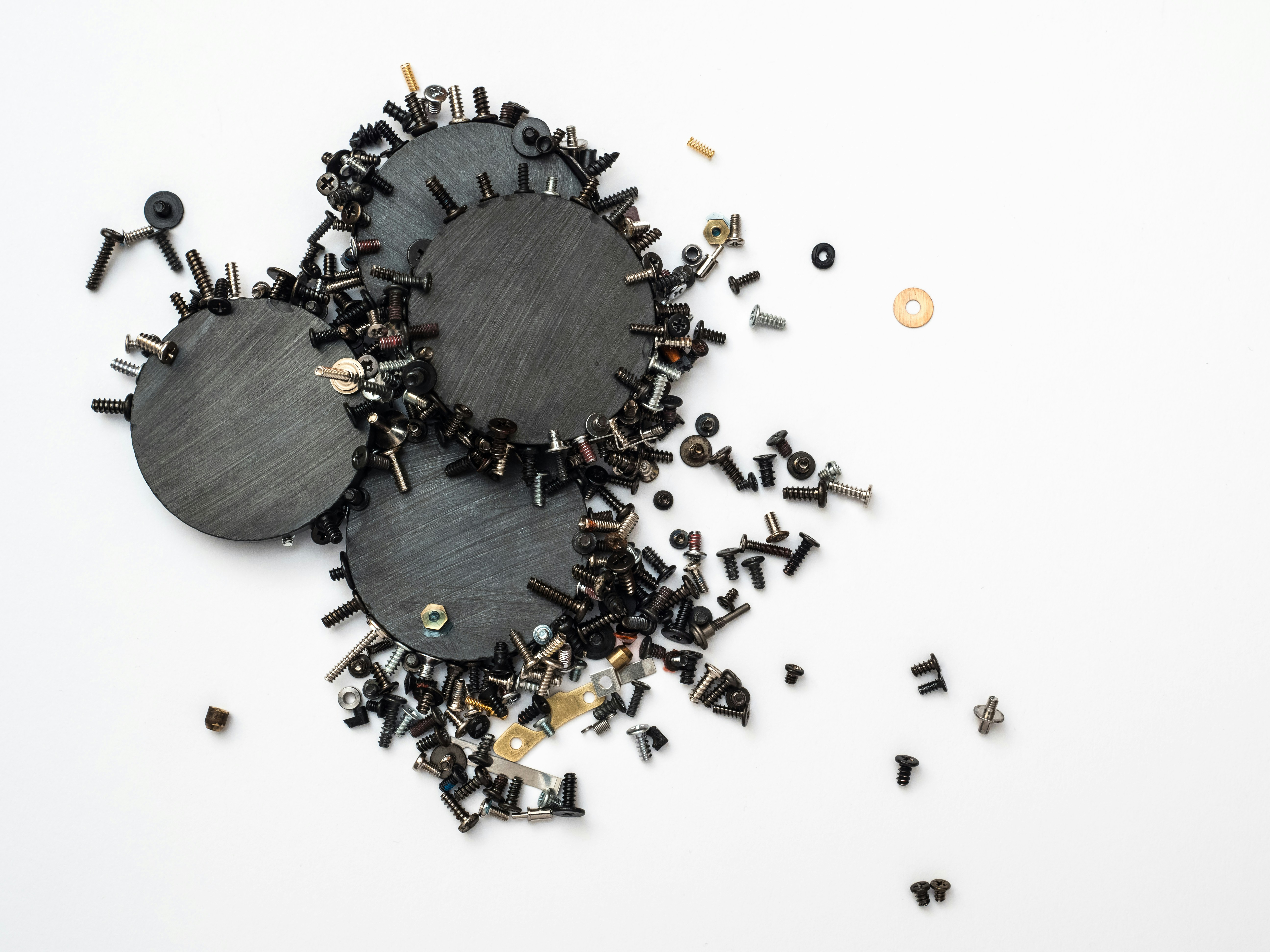Yes
No
Gravity is a force that attracts objects to each other.yes
no
In order for the box to move, which of the following need to happen?The applied force must be greater than the force of friction.
The force of friction must be greater than the applied force.
I want to move the box. If the mass of the box increases:The applied force must also increase.
The amount of applied force needs to be less.
Which of following statements describes what needs to happen in order to move the box?less friction
more friction
less applied force
Find the net force on the object, I would _________ the forces.Subtract
Multiply
Divide
To calculate the net force, I would ______ the forces.subtract
multiply
divide
Forces come in pairs.yes
no
If an object is moving at a constant rate (not changing), a balanced force is taking place.Yes
No
This image shows an unbalanced force (the ride is changing speed and direction).no
yes
If you were to travel to another planet, your mass would change.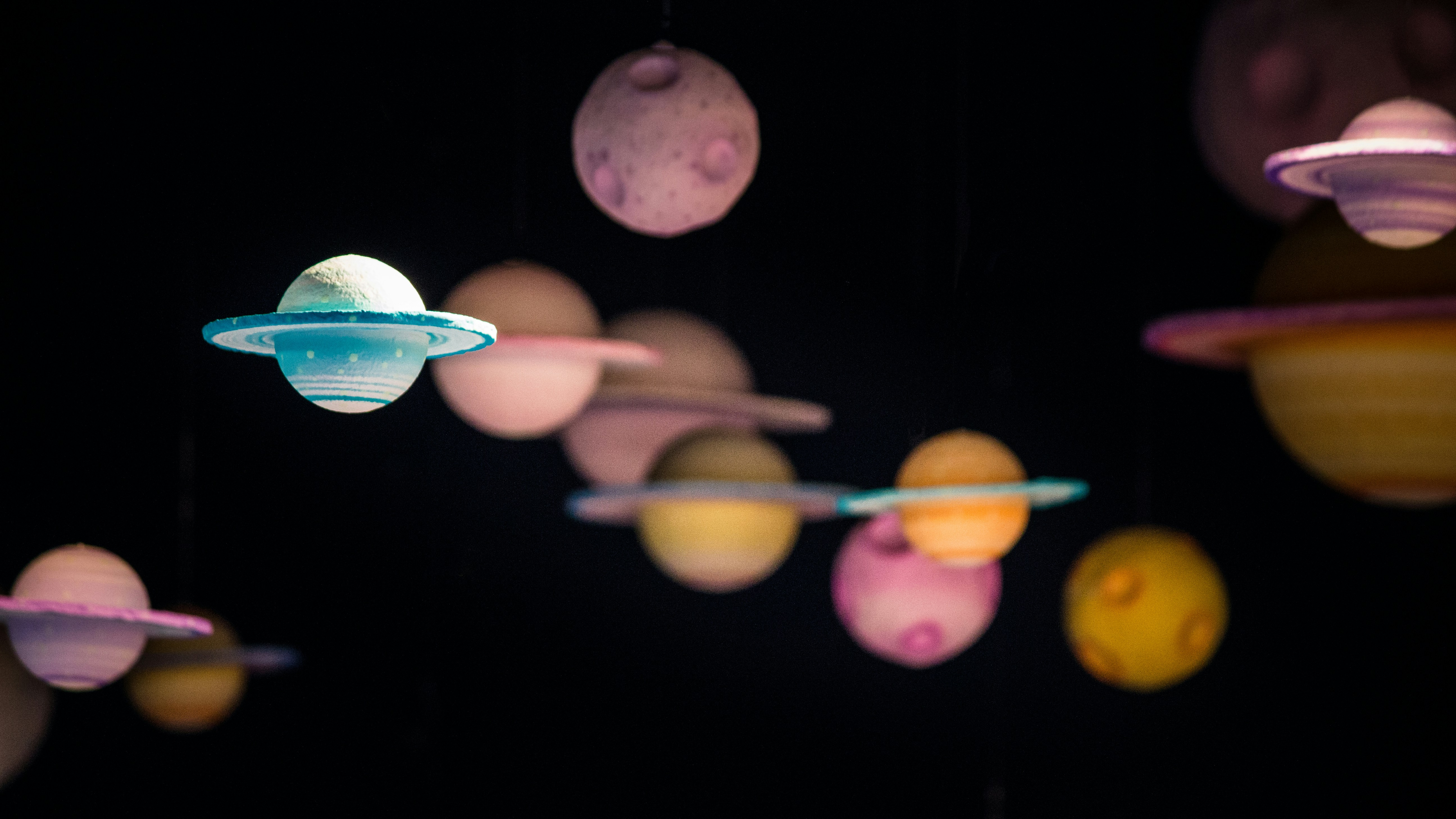No
Yes
If you traveled to another planet, your WEIGHT would change.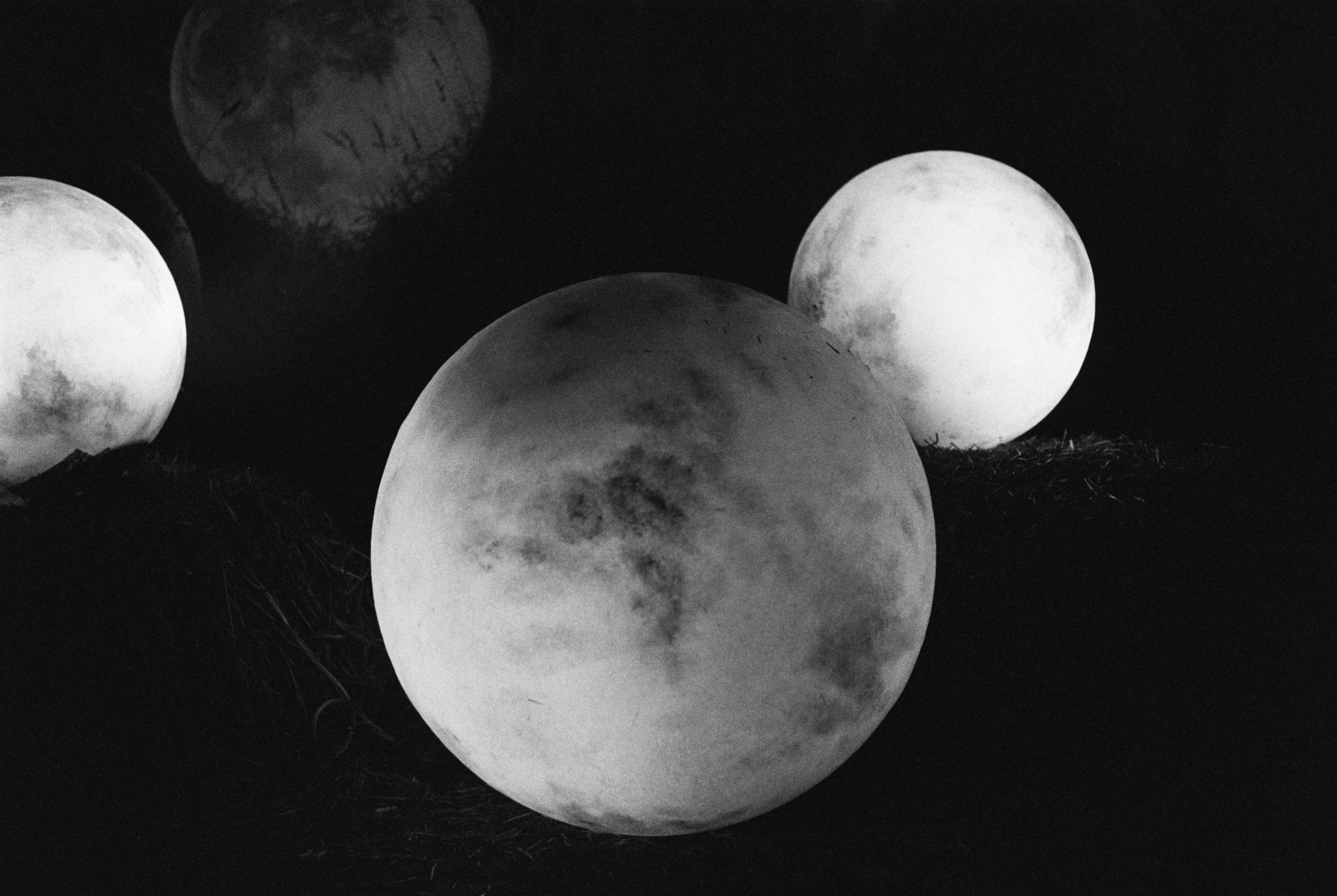yes
no
An apple falling is experiencing: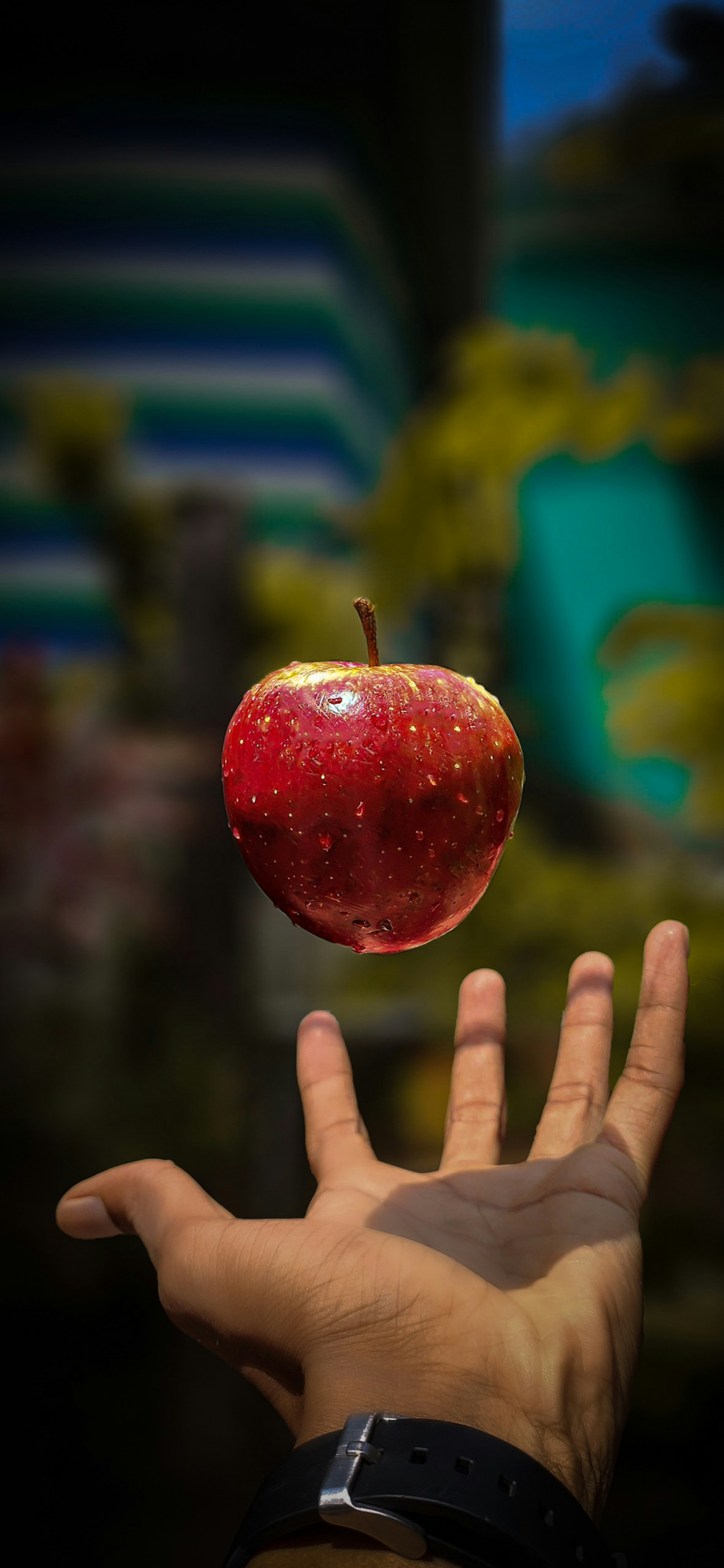Static Friction
Fluid Friction
Sliding Friction
Rolling Friciton
Which force can be useful for slowing us down?Friction
Applied
Gravity
Magnetic
The dog's kayak is experiencing:Static Friction
Fluid Friction
Rolling Friction
Sliding Friction
If the forces applied are equal and opposite in direction, which of the following will happen?The object will have a change in motion.
The object will NOT have a change in motion.
The arrows in this image represent forces that are ____________.in the same direction.
in opposite directions.
In this image, if you had to calculate the net force, you would have to ______ the forces.subtract
Which force causes an object to be pulled towards the earth?Applied
Friction
Gravitational
Magnetic
What is the rate at which objects are pulled towards the earth?19.8 m/sec/sec
9.8 m/sec/sec
The dog's car is experiencing (due to the tires):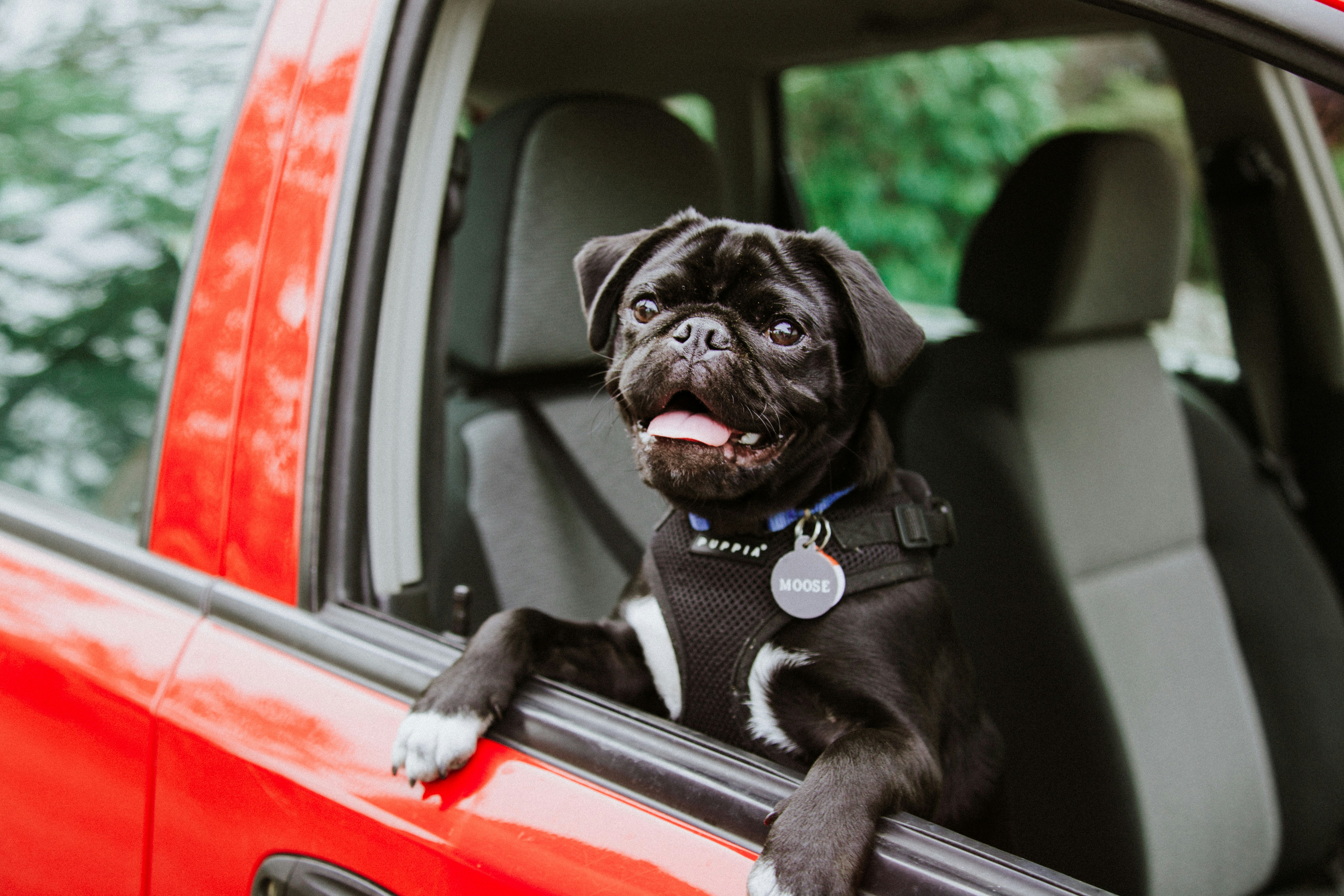Static Friction
Fluid Friction
Rolling Friction
Sliding Friction
His skateboard is experiencing: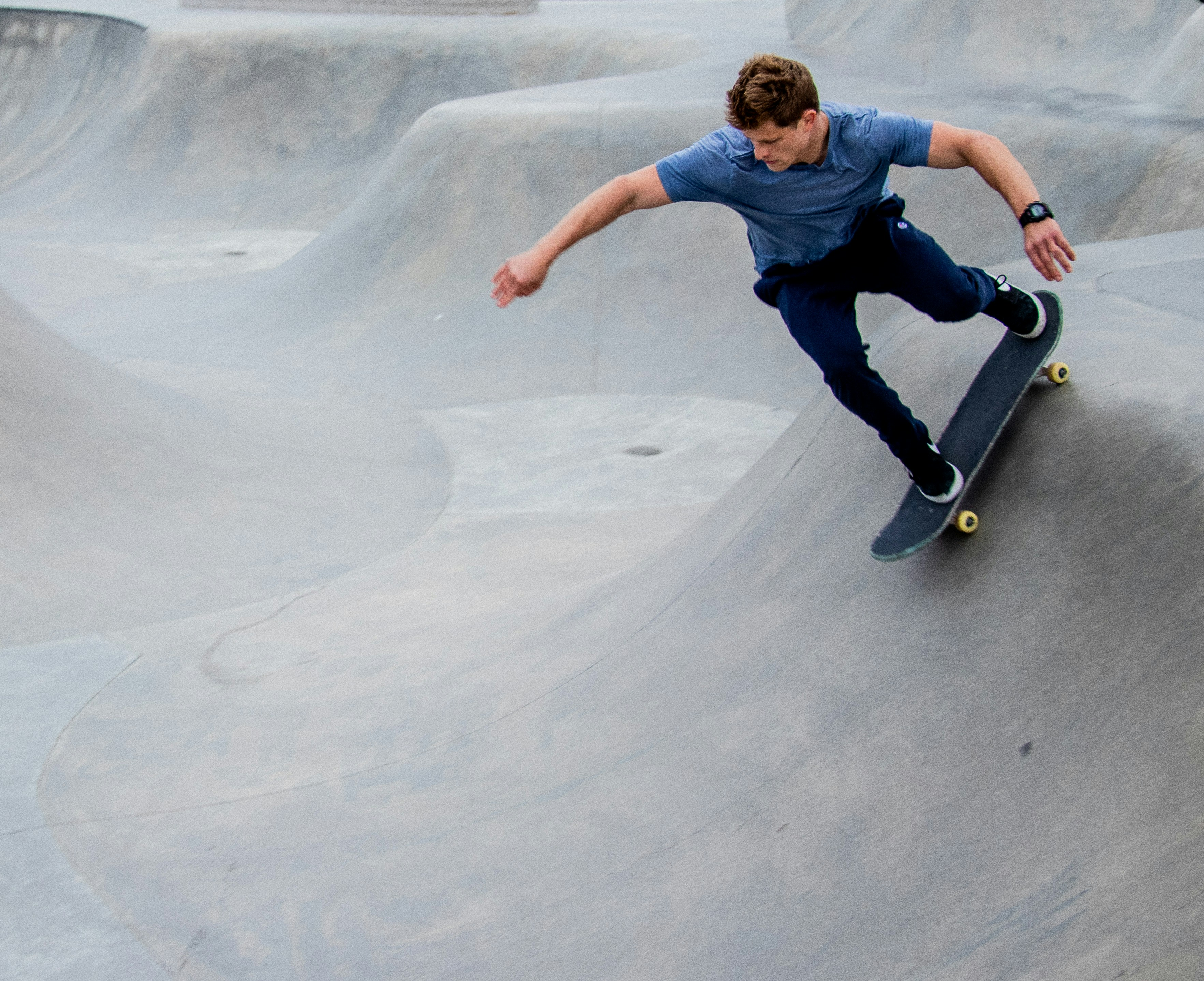static friction
rolling friction
sliding friction
fluid friction
His snowboard is experiencing: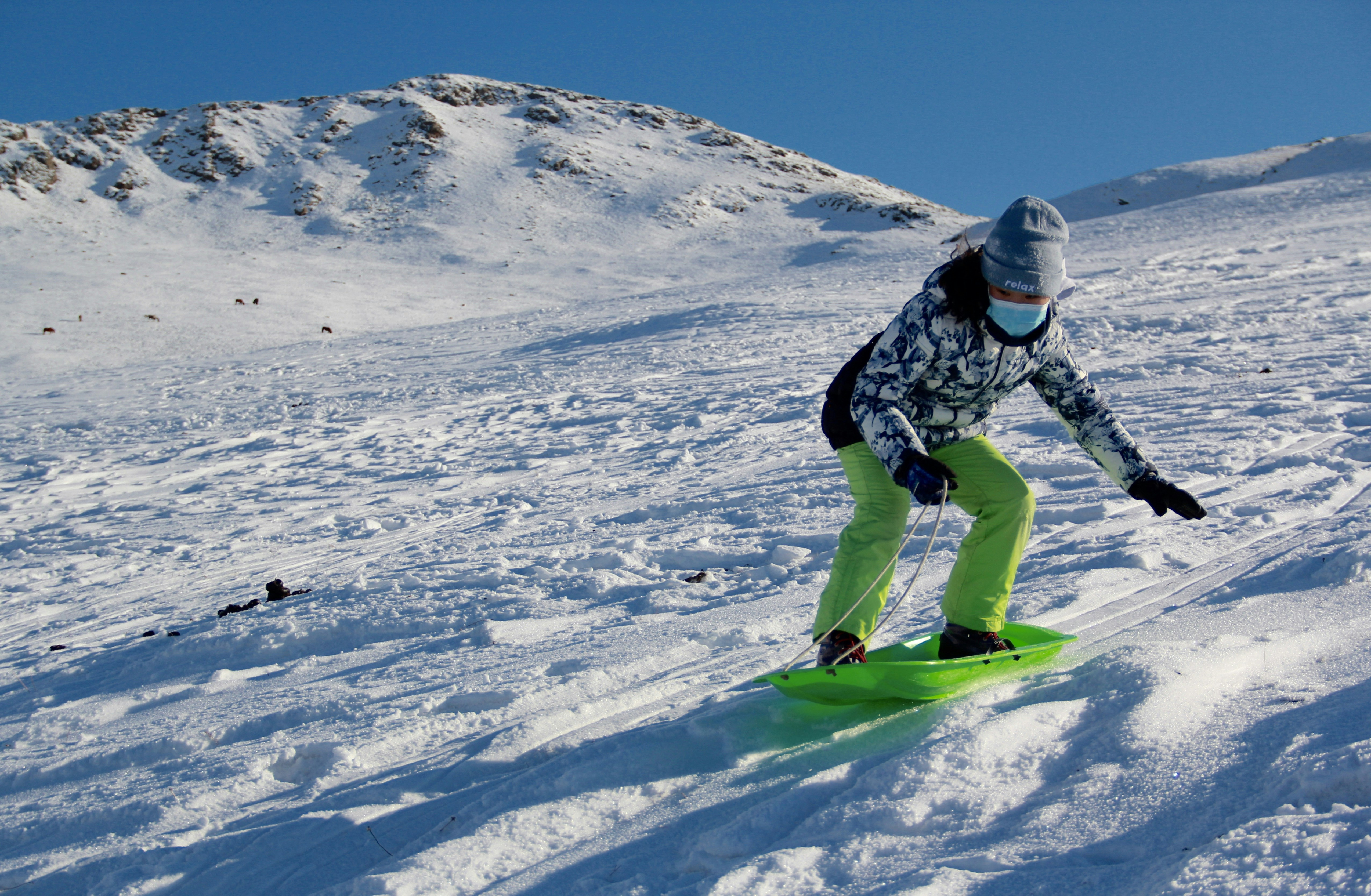static friction
rolling friction
sliding friction
fluid friction
The sea lion is experiencing what type of friction while trying to move the huge boulder: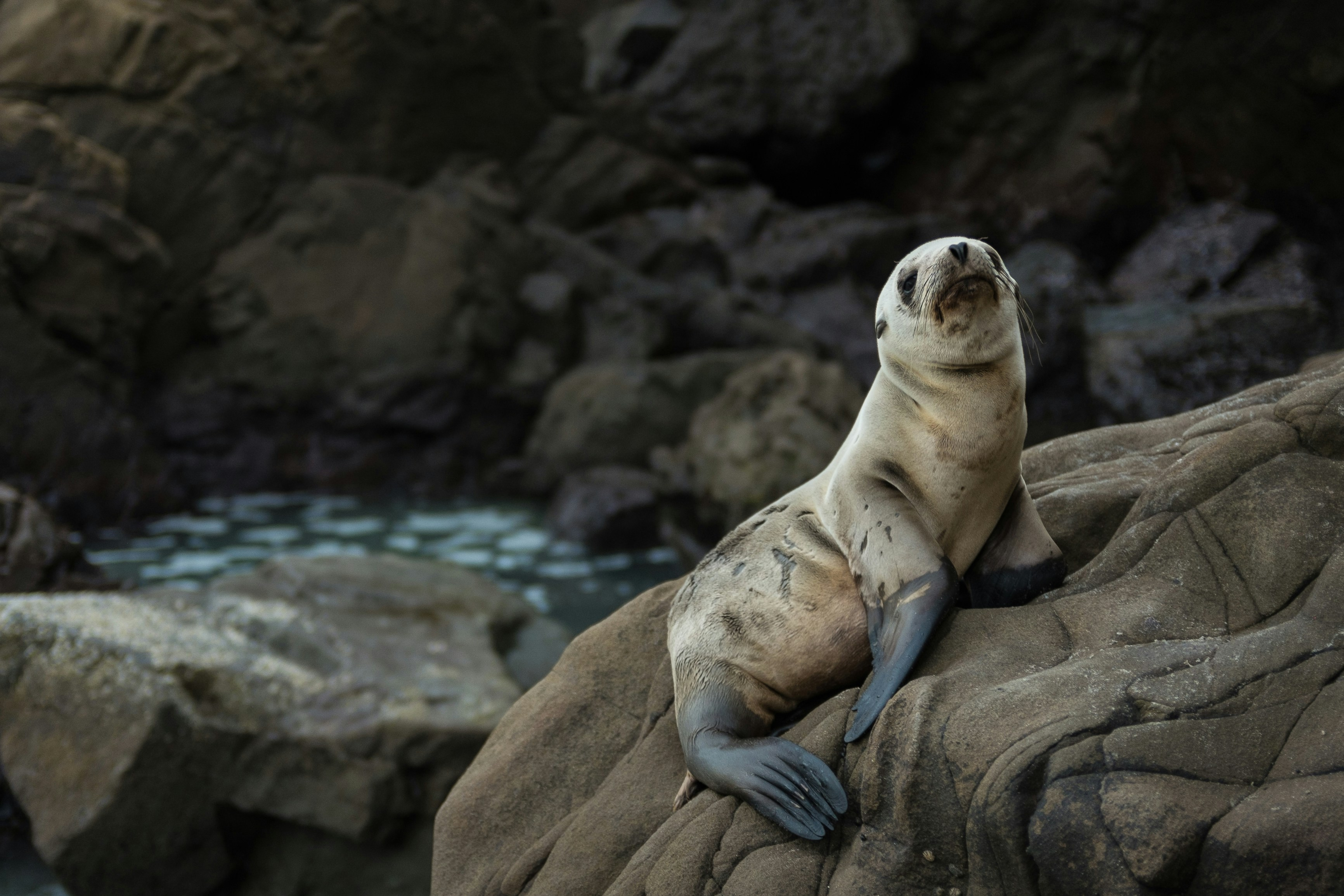static friction
rolling friction
sliding friction
fluid friction
The athlete is experiencing what type of friction while trying to get the equipment to move: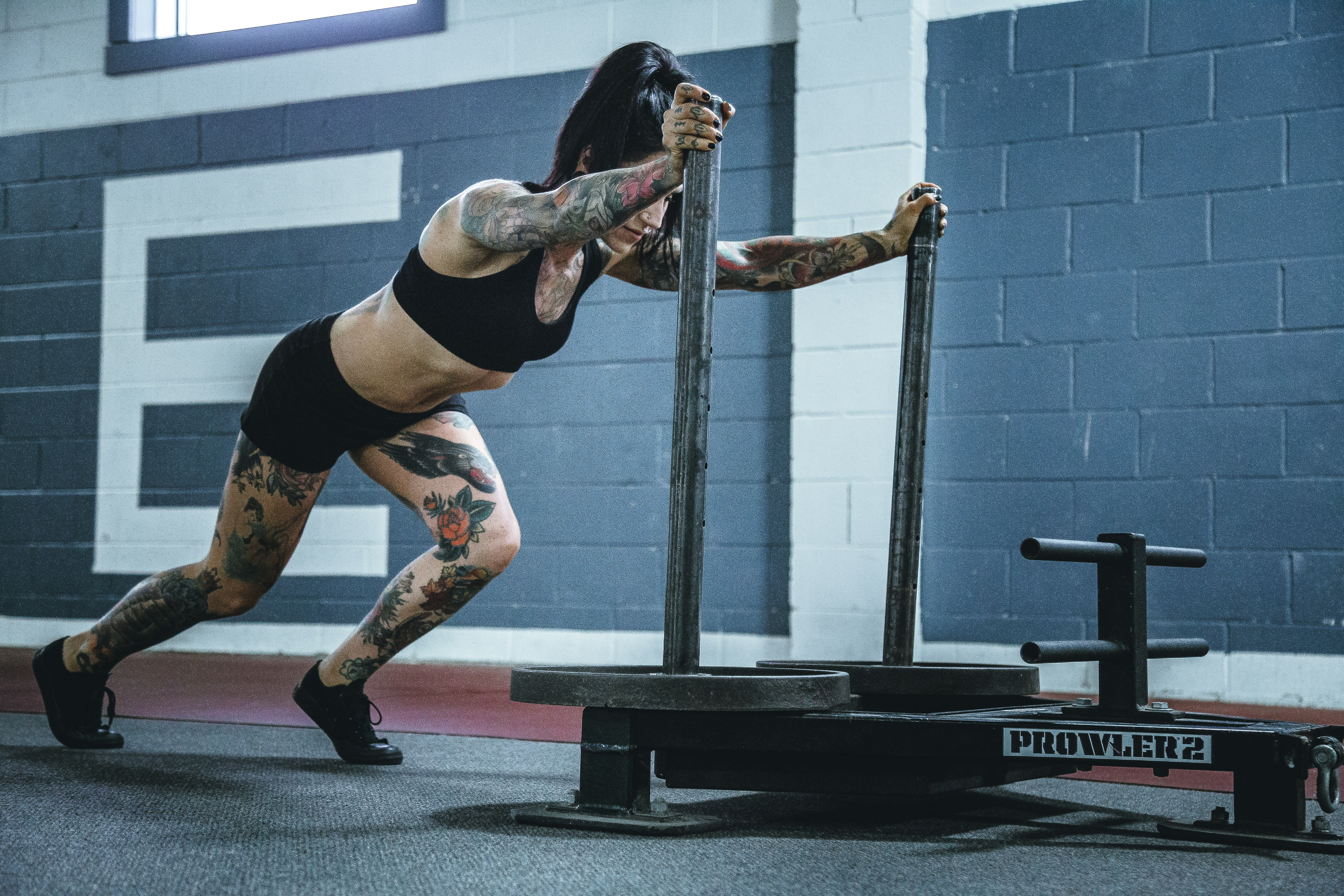static friction
rolling friction
sliding friction
fluid friction
All forces have: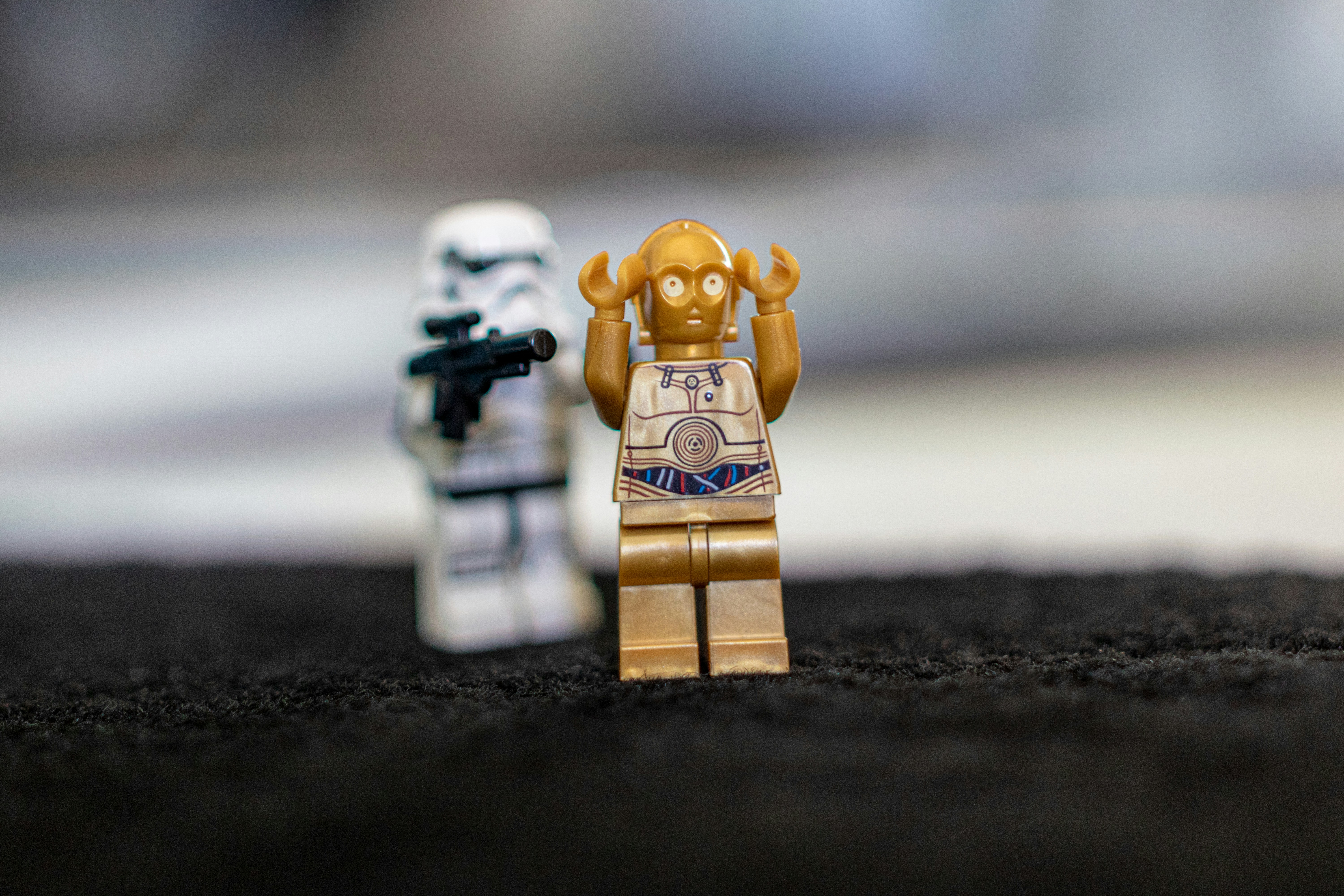magnitude and attitude
magnitude and direction
vector and direction
luke, use the force
What are 2 things that affect gravity?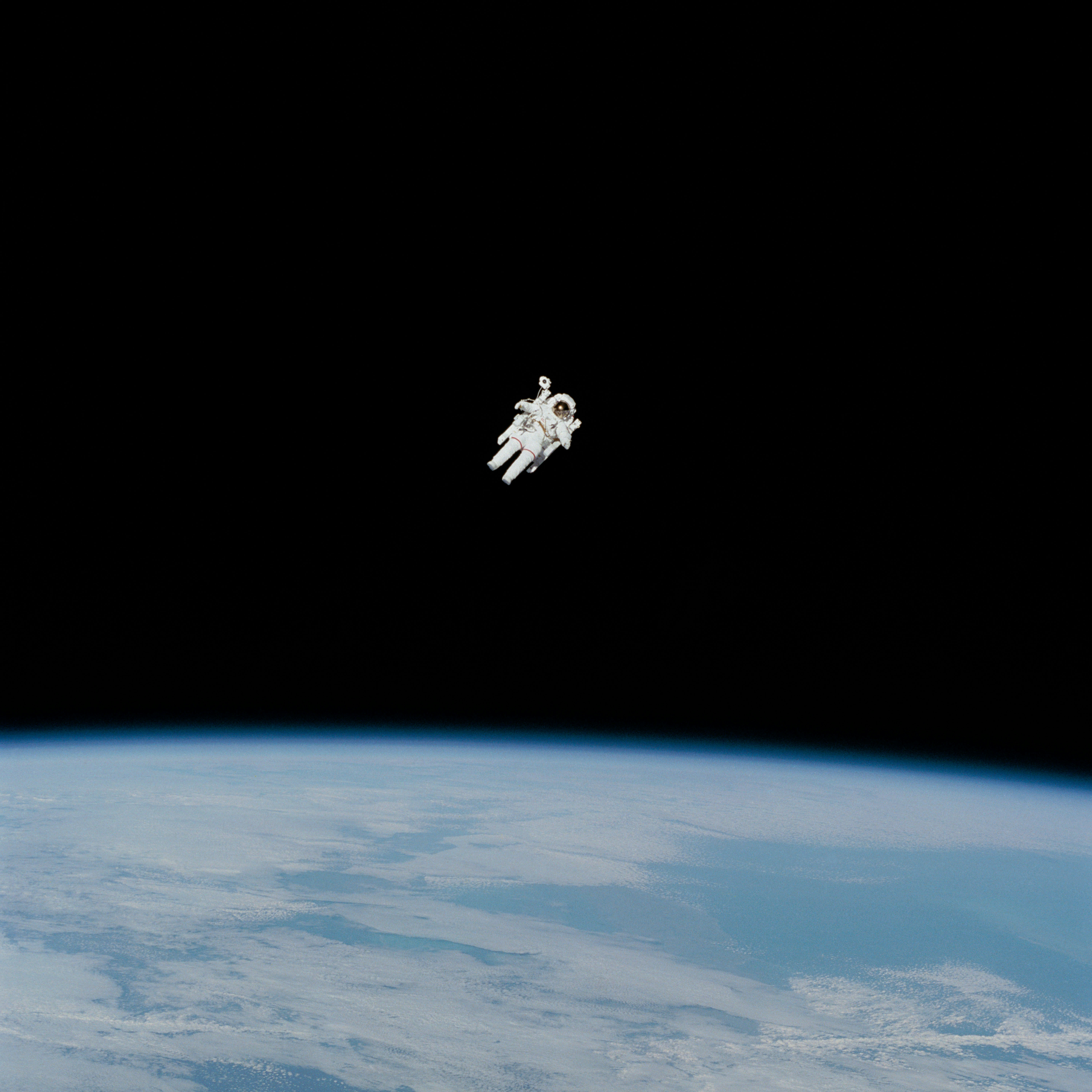weight and distance
work and tides
mass and distance
mass and direction